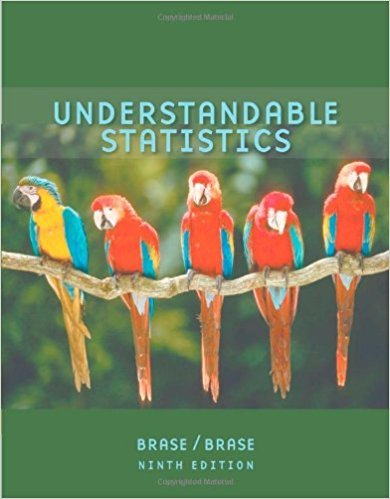×
Get Full Access to Understandable Statistics - 9 Edition - Chapter 9.1 - Problem 9
Get Full Access to Understandable Statistics - 9 Edition - Chapter 9.1 - Problem 9

×

# Dividend Yield: Australian Bank Stocks Let x be a randomISBN: 9780618949922 213

## Solution for problem 9 Chapter 9.1

Understandable Statistics | 9th Edition

• Textbook Solutions
• 2901 Step-by-step solutions solved by professors and subject experts
• Get 24/7 help from StudySoup virtual teaching assistantsUnderstandable Statistics | 9th Edition

4 5 1 388 Reviews
15
1
Problem 9

Dividend Yield: Australian Bank Stocks Let x be a random variable representing dividend yield of Australian bank stocks. We may assume that x has a normal distribution with A random sample of 10 Australian bank stocks gave the following yields. 5.7 4.8 6.0 4.9 4.0 3.4 6.5 7.1 5.3 6.1 The sample mean is For the entire Australian stock market, the mean dividend yield is (Reference: Forbes). Do these data indicate that the dividend yield of all Australian bank stocks is higher than 4.7%? Use

Step-by-Step Solution:
Step 1 of 3

Confidence Interval  Sample statistic  Usually a point estimate  Used to estimate population parameter  Sample point estimate +/- margin of error Lower endpoint sample point estimate upper endpoint Margin of error Margin of error  Measure of variability associated with point of estimate at desired level of confidence  Small margin of error means higher precision  Higher desired confidence level means a larger margin of error  Lowest value in the computed confidence level =lower endpoint  Highest value in the computed confidence level= higher endpoint Confidence interval for p  Point estimate +/- margin of error Margin of

Step 2 of 3

Step 3 of 3

##### ISBN: 9780618949922

Unlock Textbook Solution